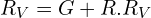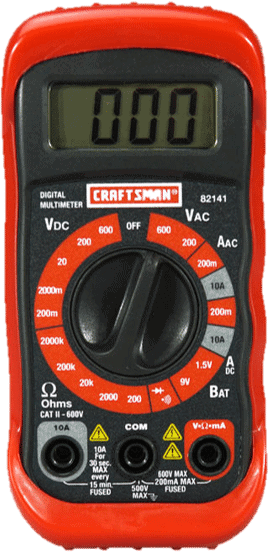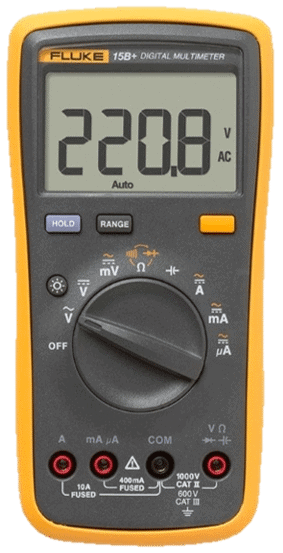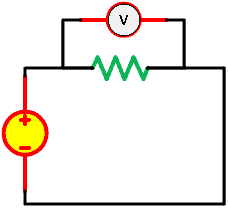# Simple Guide How to Use a Voltage MeterAfter learning how to use current with an ammeter, we will move on to the guide about how to use a voltage meter.

Contents

This guide is very straightforward, how to use a voltage meter or voltmeter for short. From its name, you know that we will use a tool to measure the voltage in a circuit. The voltmeter itself can be divided into analog and digital. The digital voltmeter alone is quite rare, it is often to use the multimeter, which has an ability to measure voltage, current, resistance, and some more.

## Voltmeter Usage

A voltmeter is an electrical measuring tool. This voltmeter can be divided into two groups, the analog voltmeter and digital voltmeter. The analog voltmeter still uses a pointer and written scale range. It also has a galvanometer along with internal resistance. The digital voltmeter often comes with a pack of measurement mode in a digital multimeter. It is capable to measure voltage, current, resistance, and more.

Just for you know, not all the voltmeters are galvanometers. It is a fact that analog voltmeter uses the same concept with a galvanometer, where we use an armature with a set of permanent magnets like what we have read in the ammeter and galvanometer.

Nowadays, digital voltmeter provides easier measurement. Digital voltmeter still uses a big resistance inside it like analog one but convert the result with analog-to-digital converter (ADC). this ADC converts any input voltage into a digital display.

Whatever voltmeter you are using, please make sure that you use it in a parallel connection with whatever you are measuring. If you are measuring the voltage in a resistor, connect the voltmeter in parallel with that resistor.

Why? You will know it below.

### Convert a galvanometer to analog voltmeter

A galvanometer can be calibrated the current or voltage in a circuit. We just need different configuration and internal resistance value to make it work correctly. When used as a voltmeter, the galvanometer needs a high resistance, connected in series.We will use the equation below:where:
G = resistance of the galvanometer
Ig = maximum deflection current of the galvanometer
V = maximum voltage
R = series-connected high resistance resistor

The effective resistance of the voltmeter can be expressed as:where the Rv will be very large, hence the voltmeter needs to be placed in parallel so it doesn’t draw all the current in the circuit. The ideal voltmeter has infinite resistance, so it doesn’t decrease the current in the circuit. Like we always know, there is no ideal condition when it comes to the practical field.

But how about the digital voltmeter? Although it doesn’t use the galvanometer, it still uses the concept of parallel connection and high resistance resistor inside it. The differences are: digital voltmeter uses resistance multiplier for the scale range and an ADC to convert the input voltage into a digital display. It is absolutely far better than the analog. You don’t need to calculate anything when using a digital voltmeter.

## How to Use a Multimeter to Test Voltage

Voltage is a potential difference between two points of an electric circuit. These two points can be nodes, branches, or circuit elements. Hence, it is safe to say that a voltmeter or voltage meter is a tool to measure the potential difference of two points of an electric circuit. It may give you some hard ideas but believe me, it is so easy after you are reading the explanation to the end.

### Step 1: Power off the circuit

As a matter of safety, we need to take caution on the electrical wiring and circuit. One simple mistake can either blow the circuit, break the components, and the worst is harming your body and even death is unavoidable. It is wise for us to turn off the circuit power first or disconnect it from the supply. We don’t know if our probes are touching the wrong points.

### Step 2: Plug the probes to the slots

You already know that a voltmeter has two probes colored red and black. We don’t need further explanation about the color since the red is assumed as ‘hot’ and black is ‘neutral’ or ‘ground’. Just put the red into the voltage (+) DC or AC and black to ground (-). The probes have a metal jack covered by plastic for you to grab them safely.

### Step 3: Turn the meter on

This can be done using a digital meter. If you are using the analog one, you don’t have any button to turn it on. We are assuming that we use digital one here.

### Step 4: Set the dial to choose the type (AC or DC)

Assume that we use a digital voltmeter or multimeter here. The multimeter has a wide range of dial. The dial is used as we use a rotary switch. The voltmeter mode has two modes, the DC and AC. The voltage DC is represented by V- and the voltage AC is represented by V~. But some multimeters put those two together in one node.

Use the rotary switch to choose the type (AC or DC) and scale range. The rotary switch in the middle can be used to switch mode for a multimeter. There will be voltage, current, and resistance with various scale ranges. Since you want to know how to use a voltmeter, turn the rotary switch to voltage mode.

If you use a multimeter that uses manual scale range, you need to know to select the right range. You can use from 2 up to 2000 VDC and 200 up to 750 VAC. If you have no idea, just select the highest one and reduce it one by one if the number display is too small. You can see it below:### Step 5: Choose the scale range

For a simple multimeter or voltmeter, there will be millivolts to a thousand volts DC and up to 750 VAC. If you are not sure about the scale, just pick the highest scale. If the result is too small you can reduce the scale one by one.

If you didn’t see any scale range, you use the auto-ranging multimeter one. You don’t need to choose the range scale, the multimeter will automatically do that job for you. You can see it below:### Step 6: Connect the probes to the circuit element’s terminal

We know that a voltage is a potential difference between two points. Connect the red and black probes on a circuit terminal or branch.

### Step 7: Read the result

Read the result in the display screen or analog pointer.

## How to Measure Millivolts

The steps about how to measure millivolts and how to measure volt are not that different. The only difference is that if we didn’t use the correct scale range, the result will be displayed as 0 volts. But worry not! The voltmeter nowadays has 1 scale range that can measure up to a few hundred millivolts. It should be enough.

The steps will be the same as before, but we only need to use the millivolts scale just as shown below:## How to Use a Multimeter to Test a Battery

Measuring a battery is very simple if you have read the guide above. Basically, you need to prepare a battery and a multimeter or voltmeter. If you use a multimeter then you need to understand the guide above. If you use a voltmeter then you just need to know how to read the scale and pointer. Moving on,

### Step 1: Prepare the battery

Prepare a battery to be measured. A small 1.5V battery will do the job.

### Step 2: Prepare your voltmeter

Both voltmeter and multimeter can do their job very well. There is no big difference between them.

### Step 3: Connect the probes to the battery

Since we only measure the voltage in a battery there is no circuit to be turned off. Just connect the red probe to the positive (+) of that battery and the black probe to the negative (-).

### Step 4: Read the result

Now we have got how much a voltage is in the battery. Since it is a small voltage battery, we don’t need to get scared for anything. Otherwise, you need to be careful when dealing with a big battery like in power generator.

## How to Use a Multimeter to Test an Outlet

When we want to measure the voltage in an outlet, we need to make sure that we have the most safety when doing it. Not only an outlet, but the high voltage measurement is also in different level from measuring electronics circuit. The supply, source, and generator voltage measurement have a huge difference on the voltage level.

The steps below have to be remembered to avoid any critical faults and injuries.

### Step 1: Turn off the switch

It is best if you can cuts off the electricity from the socket. If not, be cautious and proceed to the next step.

### Step 2: Ensure the probes are in good condition

Before starting, you need to check your multimeter’s probes. Make sure the probes are not broken, bent, or anything. Also, check their reading sensor by using ‘ohmmeter’ mode and connect those two probes together. Make sure the display shows a very small number.

### Step 3: Ensure the probes are connected in the right slots

Check the probes are correctly connected to the slots. The red probe is connected to the V~ to be able to measure AC voltage in the electric socket.

### Step 4: Set the right scale range dial

If your multimeter has auto-ranging, you can skip this step. If not, it is wise to choose the highest scale range and reduce it if the decimal in the result display is too much.

### Step 5: Connect the probes to the socket

Since the electric socket has no switch, we need to be careful here. Connect your black probe first as a neutral connection. Then connect the red probe to the ‘hot’ line. This way can prevent injuries if you connect the red probe first but your meter has a faulty. The crocodile clips are far better than jack probes, it is safer and you don’t need to grab it when measuring.

### Step 6: Turn on the electricity switch

If you skip Step 1 then you can skip this step too. Otherwise, time to switch on the electricity to the socket.

### Step 7: Read the measurement result

Nothing more to say, you can finally read the measurement result in the display.

## How to Use a Voltage Meter

Like mentioned above, the voltage meter or voltmeter needs to be connected in parallel with the circuit.

Why?

Because

A voltmeter has a very large resistance.

That explains well why we need to use it in parallel. A very large resistance in series will reduce the current in the circuit a lot. Since in the ideal voltmeter the resistance will be infinite then the current will be nearly zero. When it is connected in parallel, it will only draw a very small current. Not a big deal. You can see the image below,### What does 200m mean on a multimeter?

200 means 200 milli which we can use on 200mV or 200mA.

### What should you not do when using a multimeter?

1. Using broken probes
2. The current flow from one hand to another is very dangerous for our body and the electrical equipment
3. Connect the multimeter in parallel to measure current
4. Connect the multimeter in series to measure voltage

### What happens if voltmeter is connected in series?

In ideal condition the voltmeter has infinite resistance hence if connected in series, the current in the circuit will drop to zero.